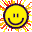終於都認清自己係咩料

#1 DutalaTortoise
16/12/21 18:25

1. Mirage (refraction of hot air)

2. Black matter is both a good absorber and good (IR) emitter

CE靠勤力，AL靠走精面，到頭來得個吉

#2 DutalaTortoise
16/12/21 18:28

1. Mirage (refraction of hot air)

2. Black matter is both a good absorber and good (IR) emitter

CE靠勤力，AL靠走精面，到頭來得個吉

syllabus一千幾百樣野，

HKEAA從來就只會翻炒或抄坊間Question Bank

#3 DutalaTortoise
16/12/21 18:41

#4 DutalaTortoise
16/12/21 18:42

#5 DutalaTortoise
16/12/21 18:56

Dialectics（辯證法）比arguing更高明

#6 DutalaTortoise
16/12/21 19:02

#7 DutalaTortoise
16/12/21 19:07

Publix goods會無哂

Social Benefits 全部唔見哂

#8 DutalaTortoise
16/12/21 19:08

Publix goods會無哂

Social Benefits 全部唔見哂

Public* goods會無哂#9 窮人唔好生仔
16/12/21 23:41

You is pure autism. People in 郊den will not have intrest on you.#10 窮人唔好生仔
16/12/21 23:43

What you talk is self speak self language without concerning other's felling. That's why people in linden fuck you.

#11 DutalaTortoise
19/12/21 18:29

What you talk is self speak self language without concerning other's felling. That's why people in linden fuck you.

#12 DutalaTortoise
19/12/21 18:30

You is pure autism. People in 郊den will not have intrest on you.

No. MK are the most authentic autists.

#13 夢追人
25/12/21 02:08#14 窮人唔好生仔
25/12/21 08:06#15 重治
25/12/21 11:45

1. Find a general expression of Sqrt[i]

2.

(c) Hence, prove that the series {1/n} diverges.#16 重治
25/12/21 11:46

1. Find a general expression of Sqrt[i]

2.

(c) Hence, prove that the series {1/n} diverges.

#17 DutalaTortoise
25/12/21 12:42

BTW, 連登太多細路，尤其是御理嗰堆

#18 DutalaTortoise
25/12/21 12:46

z=r(cosx + isinx)=re^ix

=e^(lnr + ix)=e^lnr·e^ix

i=e^[i(pi/2)]=e^[i(2npi+ pi/2)] where n is integer

sqrt(i) = i^0.5 = e^[i(2npi+ pi/2)/2]

#19 DutalaTortoise
25/12/21 12:49

integration 無與趣做

#20 DutalaTortoise
25/12/21 12:54

For the last part,

ln n is continuous for n>0.

The series {1/n} exceeds the upper bound for each ln(n), so the series does not converge.

#21 DutalaTortoise
25/12/21 13:10

integration 無與趣做

ln(r/r-1)= lnr - ln (r-1)

ln(1/r-1)= ln 1 - ln2 + ln 2 - ln3 + ... - ln(r-1)

#22 DutalaTortoise
25/12/21 13:15

1/(r-1)= -[(r - 1) - r]/(r-1)

= r/(1-r) - 1

#23 DutalaTortoise
25/12/21 16:54

1. Find a general expression of Sqrt[i]

2.

(c) Hence, prove that the series {1/n} diverges.

e^x > x + 1

equality holds iff x=0

it intersect at (0, 1)

so take the inverse function,

lnx and x-1 intersect at (1,0)

Thought inspired by Integration Bee.

#24 DutalaTortoise
25/12/21 16:55

#25 DutalaTortoise
25/12/21 17:08

1. Find a general expression of Sqrt[i]

2.

(c) Hence, prove that the series {1/n} diverges.

e^x > x + 1

equality holds iff x=0

it intersect at (0, 1)

so take the inverse function,

lnx and x-1 intersect at (1,0)

Thought inspired by Integration Bee.

BTW, e^x 一用Taylor series 爆就知大過 1+x#26 重治
25/12/21 17:09

1. Find a general expression of Sqrt[i]

2.

(c) Hence, prove that the series {1/n} diverges.

e^x > x + 1

equality holds iff x=0

it intersect at (0, 1)

so take the inverse function,

lnx and x-1 intersect at (1,0)

Thought inspired by Integration Bee.

OK seems like you make use of Macurin series of e^x, I may accept that

then you use inverse function of each function...

you kind of make use of graphs to interpret your results, which is not sound at this moment, remember this is just pure math syllabus.

and this is where you lose pp marks

If you wanna know the full steps in terms of algebra or pure math approach, try to use differentiation on f(x)=lnx-x+1#27 重治
25/12/21 17:11

For the last part,

ln n is continuous for n>0.

The series {1/n} exceeds the upper bound for each ln(n), so the series does not converge.

the word continous is wrong here

n is a natural number, which is pointwise

you need to use increasing instead

to be convergent you need to find an upper bound which is independent of n#28 重治
25/12/21 17:14

z=r(cosx + isinx)=re^ix

=e^(lnr + ix)=e^lnr·e^ix

i=e^[i(pi/2)]=e^[i(2npi+ pi/2)] where n is integer

sqrt(i) = i^0.5 = e^[i(2npi+ pi/2)/2]

#29 夢追人
25/12/21 21:20

z=r(cosx + isinx)=re^ix

=e^(lnr + ix)=e^lnr·e^ix

i=e^[i(pi/2)]=e^[i(2npi+ pi/2)] where n is integer

sqrt(i) = i^0.5 = e^[i(2npi+ pi/2)/2]

#30 夢追人
25/12/21 21:24

#31 DutalaTortoise
25/12/21 21:55

z=r(cosx + isinx)=re^ix

=e^(lnr + ix)=e^lnr·e^ix

i=e^[i(pi/2)]=e^[i(2npi+ pi/2)] where n is integer

sqrt(i) = i^0.5 = e^[i(2npi+ pi/2)/2]

#32 DutalaTortoise
25/12/21 21:59

#33 DutalaTortoise
25/12/21 22:02

For the last part,

ln n is continuous for n>0.

The series {1/n} exceeds the upper bound for each ln(n), so the series does not converge.

the word continous is wrong here

n is a natural number, which is pointwise

you need to use increasing instead

to be convergent you need to find an upper bound which is independent of n#34 重治
26/12/21 09:16

For the last part,

ln n is continuous for n>0.

The series {1/n} exceeds the upper bound for each ln(n), so the series does not converge.

the word continous is wrong here

n is a natural number, which is pointwise

you need to use increasing instead

to be convergent you need to find an upper bound which is independent of n

continuous is for function / curve, with an interval containing all values of x

series is only point by point, n=1, 2, 3, ..., you will not say n=1.2

so we call this as pointwise, not continuous

#35 DutalaTortoise
26/12/21 11:18

the word continous is wrong here

n is a natural number, which is pointwise

you need to use increasing instead

to be convergent you need to find an upper bound which is independent of n

continuous is for function / curve, with an interval containing all values of x

series is only point by point, n=1, 2, 3, ..., you will not say n=1.2

so we call this as pointwise, not continuous

#36 DutalaTortoise
26/12/21 11:30

continuous is for function / curve, with an interval containing all values of x

series is only point by point, n=1, 2, 3, ..., you will not say n=1.2

so we call this as pointwise, not continuous